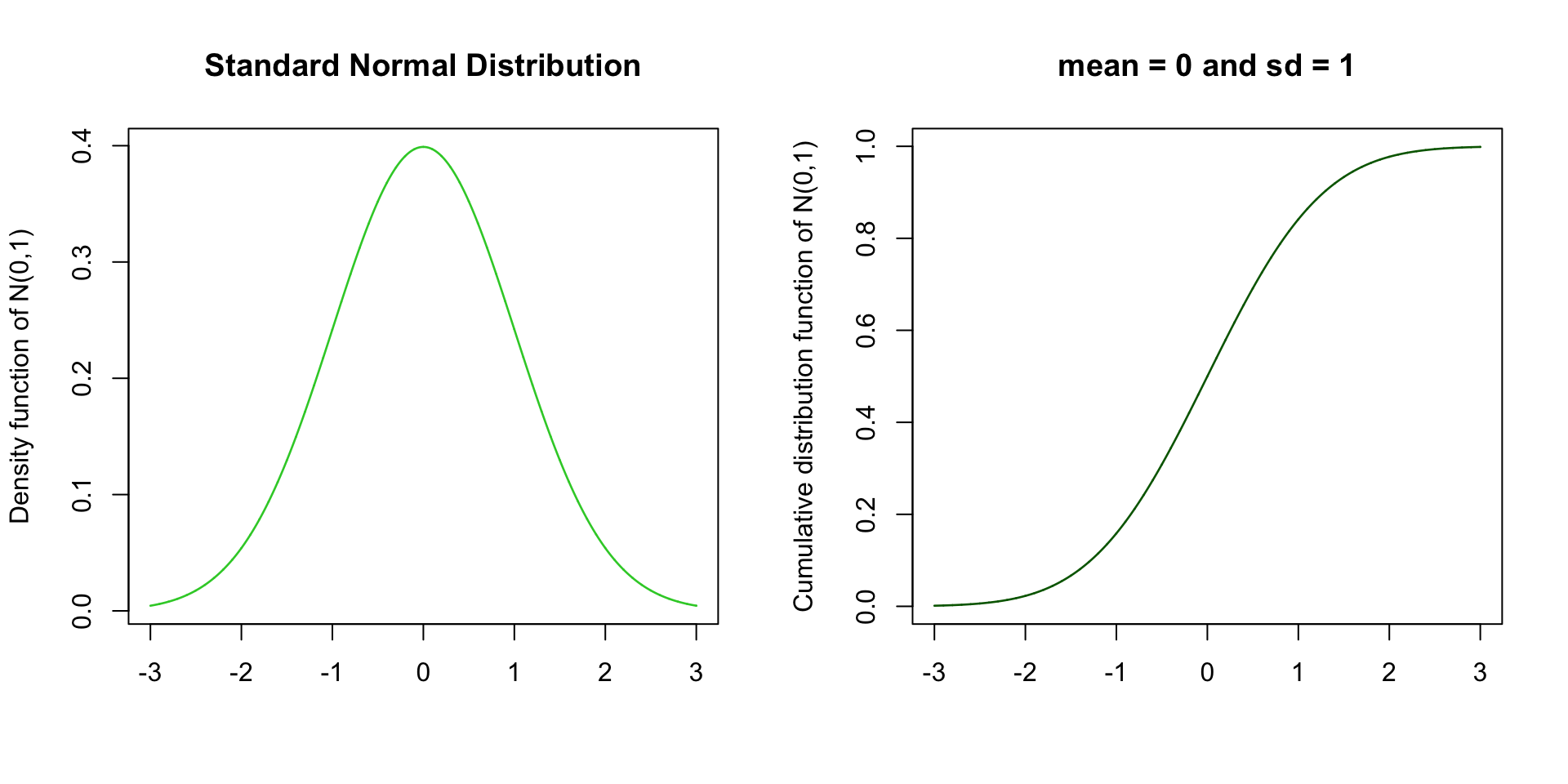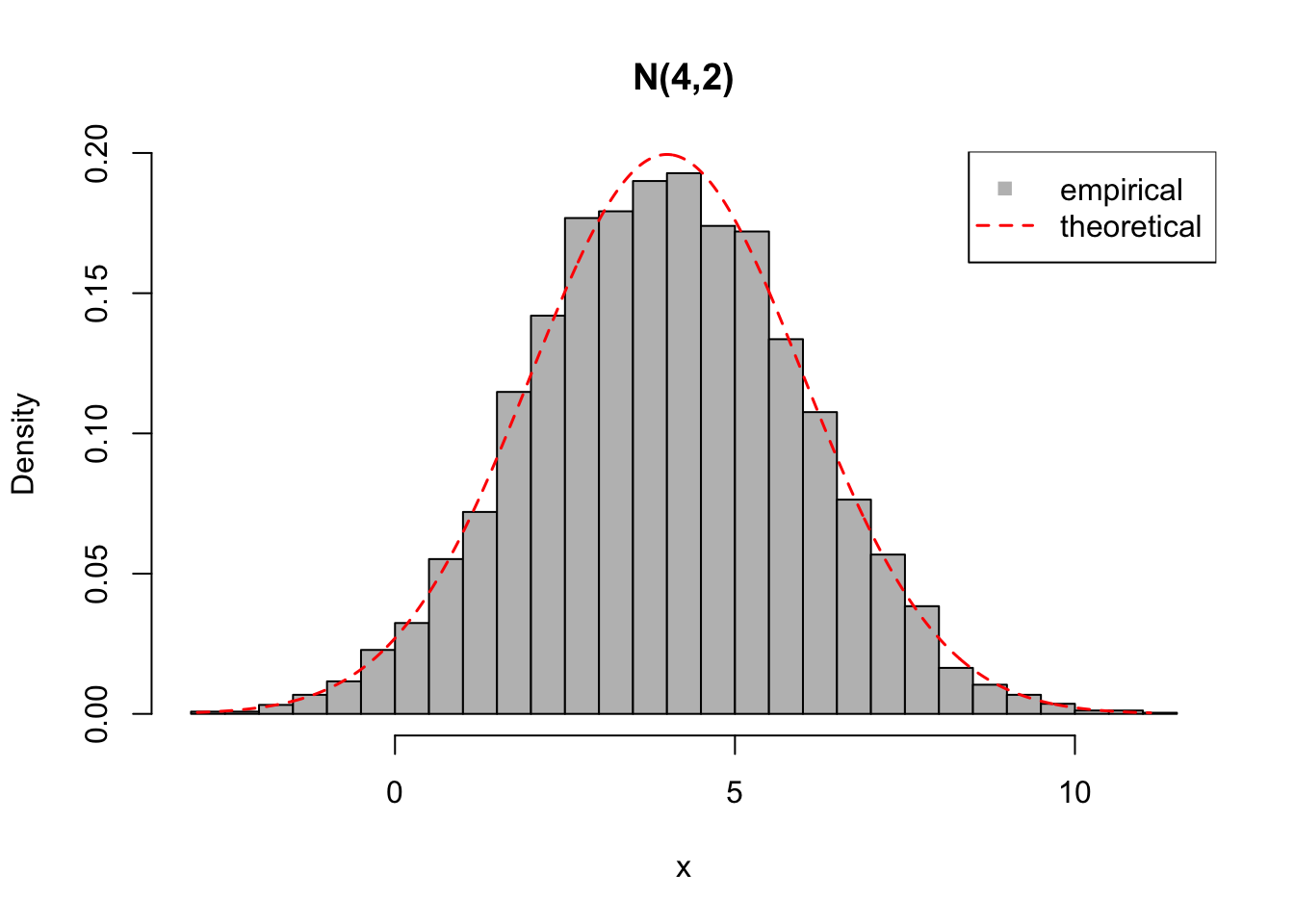During the previous lectures we got familiar with basic statistics by using the binomial distribution that models the number of “successes” in a sample of $$n$$ trials. It is a discrete distribution where the outcome variable can only take discrete values $$0,1,\ldots,n$$.

Now we move to outcome variables, such as BMI or LDL cholesterol levels, that can take any value in a continuous interval, such as, say in the interval (10.0,150.0) for BMI measured in kg/m$$^2$$.

Normal Distribution

The most prevalent continuous distribution is the Normal distribution (also called the Gaussian distribution after German mathematician Carl F. Gauss). A reason for its wide applicability is that in very many settings the distribution of sums of independent random variables tend towards a Normal distribution, and hence many complex properties, such as IQ or height or susceptibility to coronary artery disease, that result from an interplay between a huge number of individual factors both from the genome and from the environment, follow approximately a Normal distribution in the population. Read more: http://www.bmj.com/content/310/6975/298.full.

Normal distribution is defined by 2 parameters: mean (mu, $$\mu$$) and standard deviation (sigma, $$\sigma$$). Often (outside R) variance (sigma^2, $$\sigma^2$$) is used in place of standard deviation to define the second parameter. Always pay attention to which one is in question since mixing up these parameters badly mixes up all the statistics! For now, remember that the basic R functions take in standard deviation sigma.

The standard normal distribution, N(0,1), has mean = 0 and sd = 1. Let’s plot the density function dnorm(,0, 1) and the cumulative distribution function pnorm(, 0, 1) of N(0,1) next to each other. We use par(mfrow=c(1,2)) to say that we set plotting parameter mfrow to split the plotting region into 1 row and 2 columns, whence the two plots show up next to each other.

x = seq(-3, 3, 0.001) #range of x-values where we evaluate dnorm() and pnorm()
d = dnorm(x, 0, 1) #values of density function of N(0,1)
p = pnorm(x, 0, 1) #values of cumulative distribution function of N(0,1)
par(mfrow = c(1,2)) #plotting region split to 1 x 2 area to show two plots next to each other
plot(x, d, xlab = "", ylab = "Density function of N(0,1)",
main = "Standard Normal Distribution",
t = "l", lwd = 1.3, col = "limegreen")
plot(x, p, xlab = "", ylab = "Cumulative distribution function of N(0,1)",
main = "mean = 0 and sd = 1",
t = "l", lwd = 1.3, col = "darkgreen")The density plot shows that the peak is at the mean of the distribution, and the density drops from there symmetrically making a bell-shaped curve characteristic to a Normal distribution.

The cumulative distribution plot shows how the probability mass accumulates as we move from small values (here starting from -3) to larger values (here up to 3). We know that 95% of the probability mass of the N(0,1) distribution is between values -1.96 and 1.96:

qnorm(c(0.025, 0.975), 0, 1) #95% of mass of N(0,1) is between these two points
##  -1.959964  1.959964

(This fact was actually the motivation to use 1.96 as the multiplier of the SE in Lecture 3 when we derived approximate 95% confidence intervals for a proportion parameter.)

Let’s generate samples from a Normal distribution, say with mean=4 and sd=2, using rnorm() and plot the data using a histogram. Standard hist() shows on y-axis the counts of observations in each bin, but by setting prob = TRUE we can make the y-axis to scale to the values of a density function (making the total area of the histogram = 1). Then we can also show the theoretical density function dnorm(,4,2) in the same plot and compare the two.

n.samples = 5000 #samples from distribution
mu = 4 #mean
sigma = 2 #standard deviation

x = rnorm(n.samples, mu, sigma) #random sample from normal distribution
data.frame(mean = mean(x), sd = sd(x)) #show empirical mean and sd of data
##       mean       sd
## 1 3.985483 1.994015
hist(x, breaks = 40, col = "gray", prob = TRUE, main = "N(4,2)")
x.grid = seq(min(x), max(x), length.out = 1000) #grid of x-values to evaluate dnorm(, mu, sigma)
lines(x.grid, dnorm(x.grid, mu, sigma), col = "red", lwd = 1.5, lty = 2) #add dashed line to the current plot
#Let's make a legend text to appear in the top right corner
#pch is plotting symbol (15 square; -1 no symbol); lty is line type (0 no line; 2 dashed line)
legend("topright", col = c("gray","red"), legend = c("empirical","theoretical"),
lwd = 1.5, pch = c(15,-1), lty = c(0,2))We see that the histogram of 5000 random samples from N(4,2) matches quite well with the theoretical density function of N(4,2), as it should. With more samples, the match would get even tighter.

Examples 4.1

1. Generate $$n=10000$$ samples from the Normal distribution with mean 2.3 and standard deviation 3.1. Plot a histogram of the data.
x = rnorm(10000, 2.3, 3.1)
hist(x, main = "", xlab = "", col = "black", breaks = 50)